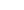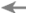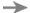# Hypothesis

A hypothesis is a testable explanation of cause and effect.

A scientific hypothesis must be a testable hypothesis. Hypotheses that cannot be tested, such as cause and effect attributed to a supernatural being or an invisible fifth dimension that cannot be detected, are not part of science. They are pseudo science.

A good hypothesis is a productive one. A productive hypothesis can:

Be easily learned and applied

Explain the past accurately and persuasively

Make accurate predictions about the future

Generate new even more useful hypotheses

Be applied to a wide variety of situations

Be easily tested

The more of these attributes a hypothesis has, the more problems it can solve.

## Why this abstraction is important for solving difficult problems

The Scientific Method

1. Observe a phenomenon that has no good explanation.

2. Formulate a hypothesis.

3. Design an experiment(s) to test the hypothesis.

4. Perform the experiment(s).

5. Accept, reject, or modify the hypothesis.

The second step in the Scientific Method is to formulate a hypothesis. Here the challenge is to synthesize the most productive hypothesis possible.

Problem solvers utilize a constant stream of hypotheses. Some are reused. The rest are new. A problem is solved by the combination of hypotheses that led to its solution. The more hypotheses you can reuse when solving a problem, the greater your chance of success and the faster and more efficiently you will solve the problem.

The best way to maximize hypothesis reuse is to use a mature problem solving process. A process is a reusable series of steps and related practices for achieving a goal, such as solving a class of similar problems. Each step is a reusable hypothesis of what will help you the most in the many steps required to achieve the goal.

What process are you using to solve your biggest problems? How well is it working? What's the percentage of hypotheses that you have to work out on your own, out of the total number needed to solve the problem? The higher this is, the harder the problem is to solve.

Browse the GlossaryPreviousNextRELATED INFORMATION
Experimentation

Hypotheses are tested by experimentation. Thus a good hypothesis is designed to be easily tested. Ideally it makes a specific prediction that is easily measured, with a narrow, easily controlled set of preconditions for the prediction to hold.